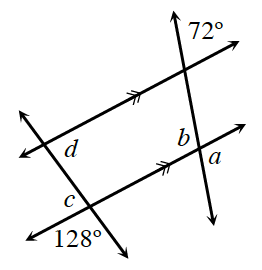Home > GB8I > Chapter cc43 > Lesson cc43.3.3 > Problem3-139

3-139.

Examine the diagram at right. Then use the information provided in the diagram to find the measures of angles $a, b, c,$ and $d$. For each angle, name the relationship that helped justify your conclusion. For example, did you use vertical angles? If not, what type of angle did you use? Homework Help ✎

Review the Math Notes box in Lesson 1.2.1.